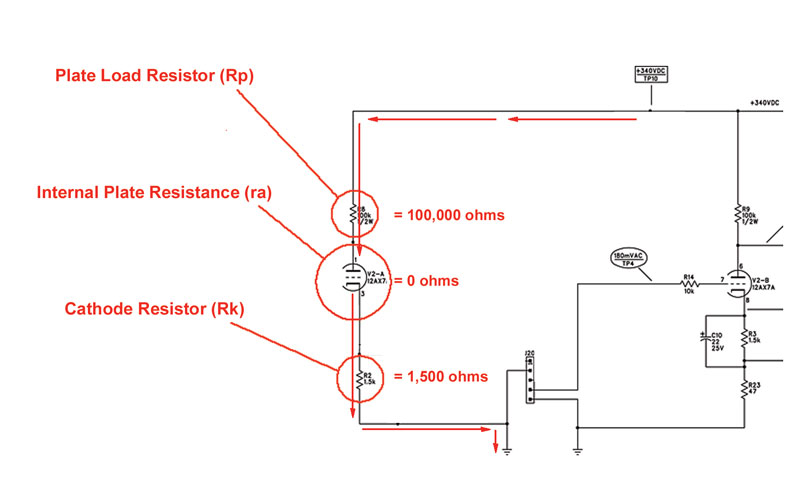## Monday, March 2, 2009

### Fender Champion 600 Preamp Bias Part 2b

In Preamp Bias Part 2a we derived this load line for the first 12AX7 gain stage:To find the maximum current we imagined that the full preamp B+ voltage was across the plate resistor. This post is an addendum to for those interested in why that is a reasonable way to estimate the maximum current. First we should look as the path of the current from the B+ power supply to ground:From the 340VDC power supply the current flows through the plate resistor, on through the tube itself and then through the cathode resistor to ground.* So here's what he circuit "looks like" for DC current flow:So there are three separate resistances to the flow of current. All three are in series so the total resistance will be all three of those resistance added together. Using Ohm's law again:The total current flowing in the circuit will be 340 volts divided by the the total of those three resistances.

From both Ohm's law and from intuition we know that more resistance will mean more opposition to current flow. Hence less resistance will mean more current flow.

So to find the maximum current flow we want to be thinking of the condition under which the circuit has the least possible resistance.

This means that we have to make all three of those resistances as small as possible. The plate resistor (Rp) is a fixed value of 100,000 ohms - nothing we can do about that.

The cathode has a fixed resistor too (Rk) - 1500 ohms. It's value is not going to change either.

The internal plate resistance (ra) is the resistance the tube itself contributes to the circuit. This value varies very widely - practically from zero to infinity. This change in resistance is actually key to it's functioning as an amplifier.

We want to be calculating the point at which the total resistance is smallest. Since that will occur when the tube's internal resistance is smallest we'll use the smallest possible internal resistance in our calculation. That makes things easy. That's effectively zero.

So that leaves us with the following:That means the total resistance is just the sum of the plate resistor value and the cathode resistor value.

But in Part A I said all we used to calculating the maximum current is the plate resistor value. Now we're using the cathode resistor too. Why the change?

There's no change really. The cathode resistor is very small compared to the plate resistor - only 1.5% of the value so we can ignore it for the calculation. If you're designing a stage from scratch you won't pick the cathode value until after you've drawn the load line so it's easiest to be in the habit of ignoring it for the common stages you'll find in most guitar amps.

* Capacitors block DC. Since we're looking at the DC current flow, no current will be flowing through any of the capacitors.